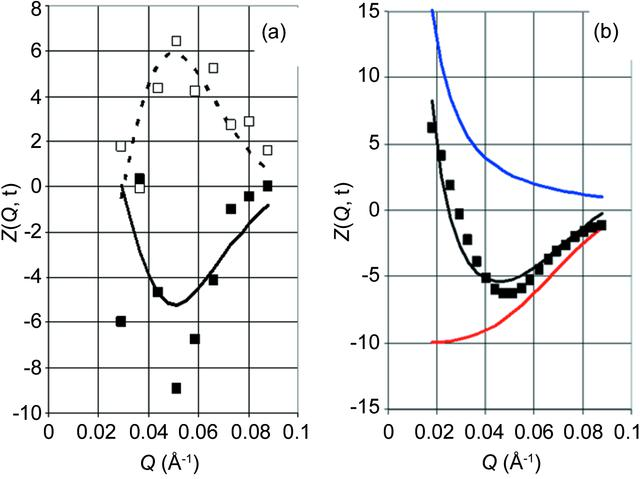disable zoom     view article Figure 9 Time-resolved neutron scattering Zexp(Q, t) for sample 1 versus Q. (a) At t = 2.5 s and 7.5 s in the lower and upper part of the figure, respectively. Symbols represent experimental data averaged over −1 < t < 1 s and −0.01 < Q < 0.01 Å−1, and the lines are the experimental data after two-dimensional Fourier analysis. (b) The filled black squares indicate Zexp(Q, t) after two-dimensional Fourier analysis at t = 2.5 s and the solid black line denotes Zcalc(Q, t) at t = 2.5 s. Also shown is the decomposition of Zcalc(Q, t) into SANS (solid red line) and background (solid blue line). The units of the intensity are as in Fig. 5.

IUCrJ
Volume 3| Part 5| September 2016| Pages 326-340
ISSN: 2052-2525